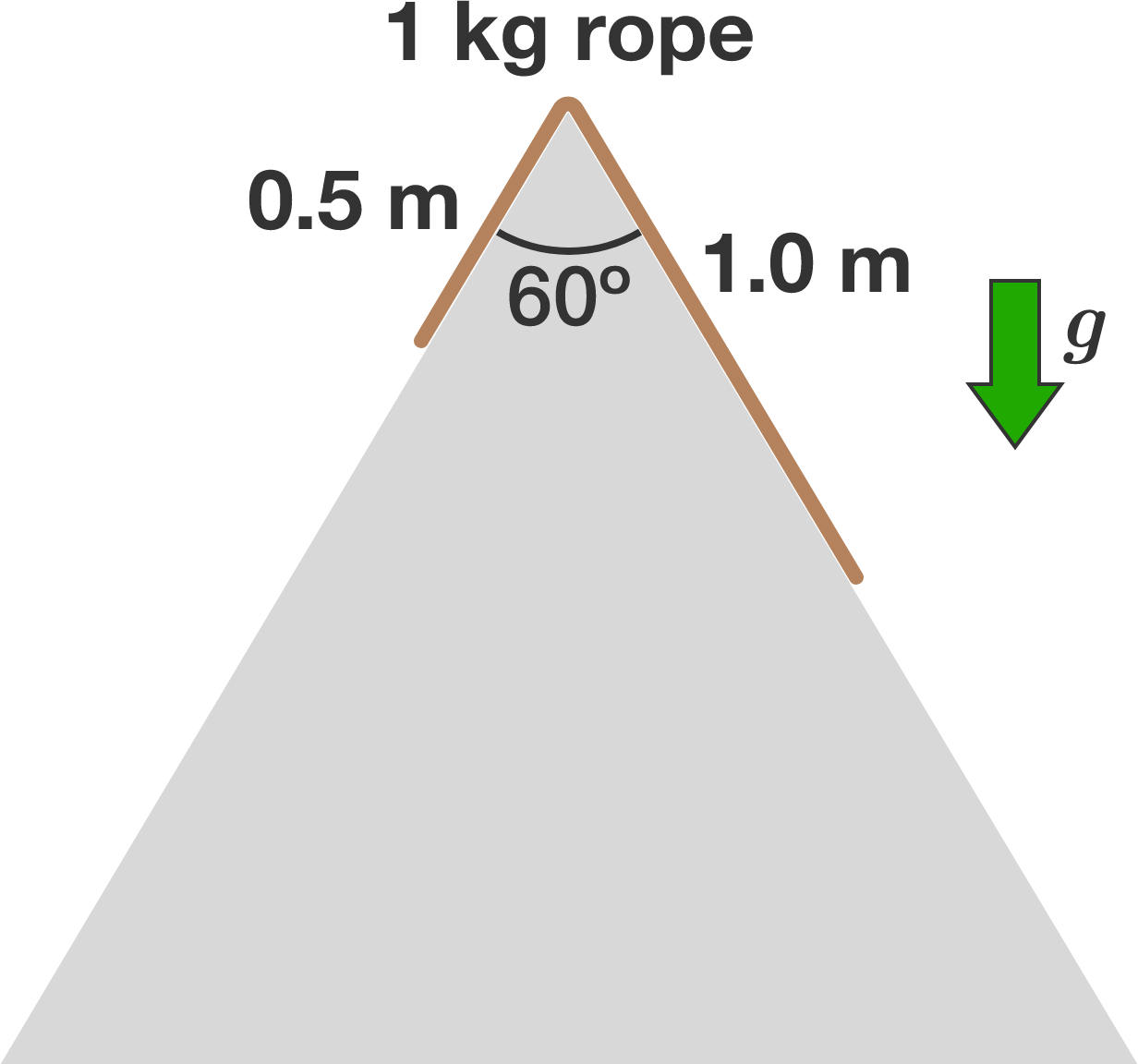# Rope Sliding on Double RampA $\SI{1}{\kilo\gram}$ rope of length $\SI{1.5}{\meter}$ slides down a frictionless double ramp in the shape of an equilateral triangle, as shown in the diagram above. At time $t = 0$, the rope is at rest with $\SI{1}{\meter}$ of its length on the right side of the ramp.

How much time (in seconds, to 3 decimal places) elapses before all of the rope is on the right side?


Details and Assumptions:

• The downward gravitational acceleration is $\SI[per-mode=symbol]{10}{\meter\per\second\squared}.$
×

Problem Loading...

Note Loading...

Set Loading...